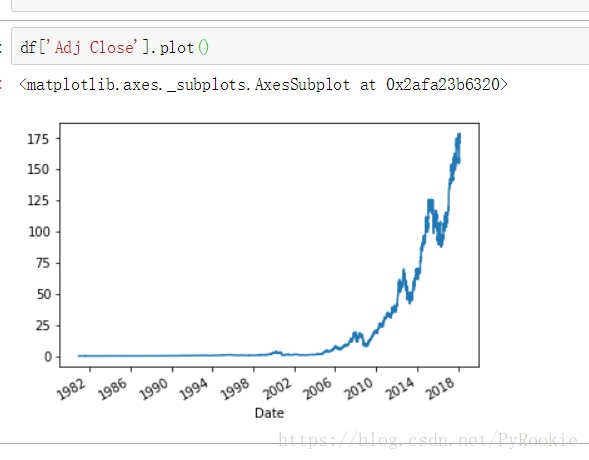﻿ Python高级数据分析之pandas和matplotlib绘图_python_脚本之家

# Python高级数据分析之pandas和matplotlib绘图

更新时间：2022年05月14日 10:04:16   作者：PyRookie
Matplotlib是一个强大的Python绘图和数据可视化的工具包,下面这篇文章主要给大家介绍了关于Python高级数据分析之pandas和matplotlib绘图的相关资料,文中通过示例代码介绍的非常详细,需要的朋友可以参考下

## 一、matplotlib 库

`import matplotlib.pyplot as plt`

```>>> jin = plt.imread("./jin.png")
>>> jin
array([[[0.24313726, 0.24313726, 0.24705882],
...,

[0.7294118 , 0.7294118 , 0.7294118 ]]], dtype=float32)

>>> jin.shpae
(273, 411, 3)
'''

'''
```

2）plt.imshow(ndarray)显示图片

```>>> plt.imshow(nd)  #将加载后的多维数组传入就可以将图片渲染出来
'''

'''
```

3）plt.imsave(ndarray)保存图片

`plt.imsave(ndarray)`

```plt.imsave('图片名称.png',图片数据,cmap='gray')    #保存图片
cmap:将图片保存为黑白图片
```

## 二、Pandas绘图

### 1.绘制简单的线型图

#### 1.1）简单的Series图表示例 .plot()

```# 正弦曲线
x = np.linspace(0,2*np.pi,100)      # 从0 到 2π  取100份
y = np.sin(x)                       # y 为sin(x) 的值
s = Series(data=y,index=x)          # 构建一个Series对象
s.plot()                  # 使用Series的plot()方法```#### 1.2) 两个Series绘制的曲线可以叠加2）简单的DataFrame图表示例 .plot()

```x = np.linspace(0,2*np.pi,100)          # 从0 到 2π  取100份
df = DataFrame(data={'sin':np.sin(x),'cos':np.cos(x)},index=x)   #创建DataFrame对象
df.plot()```### 2.数据驱动的线型图(分析苹果股票)

```import numpy as np
import pandas as pd
from pandas import Series,DataFrame```

1.读取数据

```df = pd.read_csv('./data/AAPL.csv')2.检查数据类型

df.dtypes

Date          object       #时间的数据类型为对象,在使用中需要做处理
Open         float64
High         float64
Low          float64
Close        float64
Volume       float64
dtype: object

3.将’Date’这行数据转换为时间数据类型

pd.to_datetime(Series对象)

```df['Date']  # 这一列获取出来是一个Series
type(df['Date'])
df['Date'] = pd.to_datetime(df['Date'])```

df.dtypes

Date         datetime64[ns]  #此时已经是时间类型的数据了
Open                float64
High                float64
Low                 float64
Close               float64
Volume              float64
dtype: object

4.将’Date’设置为行索引

```df.set_index('Date',inplace=True)
'''
inplace:改变原来的变量的值
'''
```

`df['Adj Close'].plot()`### 3.绘制简单的柱状图

1) Series柱状图示例,kind = ‘bar’/’barh’

```'''
data:  生成之后为Y轴的数据
index: 生成之后为X轴的索引
'''
s = Series(data=np.random.randint(0,10,size=5),index=list('abcde'))  #随机产生5个数
s.plot(kind='bar')``````'''
data:  生成之后为Y轴的数据
index: 生成之后为X轴的索引
'''
s = Series(data=np.random.randint(0,10,size=5),index=list('abcde'))
s.plot(kind='barh')```2) DataFrame柱状图示例

```'''
index: 生成数据X轴的索引
columns: 特征的名称
'''
df = DataFrame(np.random.randint(0,10,size=(8,4)),index=list('abcdefgh'),columns=list('ABCD'))
df.plot(kind='bar')```### 4.绘制简单的直方图

```s = Series(np.random.normal(loc=0,scale=5,size=10000))    # normal 正态分布
s.hist()
#这里模拟出一组以0为中心,标准差为5的正态分布数据, 绘制出的直方图如下```### 5.绘制简单的核密度(“ked”)图

```Series(np.random.normal(loc=0,scale=5,size=10000))
s.plot(kind='kde')``````ndarr1 = np.random.normal(loc=-10,scale=5,size=5000)  #第一组数据
ndarr2 = np.random.normal(loc=15,scale=2,size=5000)  第二组数据
ndarr = np.concatenate([ndarr1,ndarr2])   #将两组数据合为一组
s = Series(ndarr)    #生成Series对象
s.plot(kind='kde')  # 核心 密度 估计 用来展示某个位置出现内容的概率的估计值
s.hist(density=True)  # density表示直方图也以 密度概率的值来展示  hist用来展示某个位置出现内容的实际频率
#展示效果如下```
```'''
s.plot(kind='kde')  展示某个位置的估计值
s.hist(density=True)  表示某个位置出现内容的实际频率
'''```### 6.绘制简单的散点图

```df = DataFrame(np.random.randint(0,150,size=(20,3)),columns=['python','math','eng'])
df   #模拟一个DataFrame数据，列名为'python','math','eng'

python  math    eng
0   141     41      55
1   37      76      96
2   61      28      135
。。。
17  124     103     83
18  86      47      44
19  35      85      85```

```df.plot(kind='scatter',x='python',y='eng')
# kind = 'scatter'  ， 给明标签columns``````np.random.randint(-10,10,1)   # 0-10之间 随机取一个数
df['php'] = df['python'].map(lambda x: x*0.9+np.random.randint(-10,10,1))
df

python  math    eng php
0   121     67      15  113.9
1   148     33      149 123.2
。。。
18  79      77      108 74.1
19  105     107     53  102.5```